Vector Calculus NAT Level - 2

# Vector Calculus NAT Level - 2

Test Description

## 10 Questions MCQ Test Topic wise Tests for IIT JAM Physics | Vector Calculus NAT Level - 2

Vector Calculus NAT Level - 2 for IIT JAM 2023 is part of Topic wise Tests for IIT JAM Physics preparation. The Vector Calculus NAT Level - 2 questions and answers have been prepared according to the IIT JAM exam syllabus.The Vector Calculus NAT Level - 2 MCQs are made for IIT JAM 2023 Exam. Find important definitions, questions, notes, meanings, examples, exercises, MCQs and online tests for Vector Calculus NAT Level - 2 below.
Solutions of Vector Calculus NAT Level - 2 questions in English are available as part of our Topic wise Tests for IIT JAM Physics for IIT JAM & Vector Calculus NAT Level - 2 solutions in Hindi for Topic wise Tests for IIT JAM Physics course. Download more important topics, notes, lectures and mock test series for IIT JAM Exam by signing up for free. Attempt Vector Calculus NAT Level - 2 | 10 questions in 45 minutes | Mock test for IIT JAM preparation | Free important questions MCQ to study Topic wise Tests for IIT JAM Physics for IIT JAM Exam | Download free PDF with solutions
 1 Crore+ students have signed up on EduRev. Have you?
*Answer can only contain numeric values
Vector Calculus NAT Level - 2 - Question 1

### Evaluate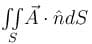where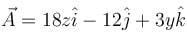and S is the part of the plane 2x + 3y + 6z = 12 which is located in the first octant.

Detailed Solution for Vector Calculus NAT Level - 2 - Question 1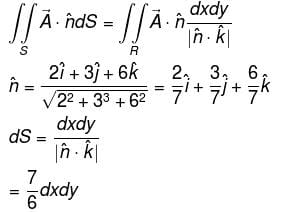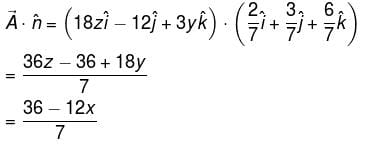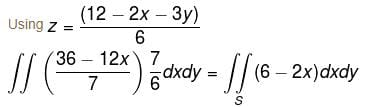= 24

*Answer can only contain numeric values
Vector Calculus NAT Level - 2 - Question 2

### Find the value of constant (a + b + c) so that the directional derivative of the function f = axy2 + byz + cz2x3 at the point (1, 2, –1) has maximum magnitude 64 in the direction  parallel to y axis :

Detailed Solution for Vector Calculus NAT Level - 2 - Question 2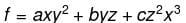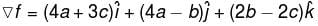lies along y axis
So, 4a + 3c = 0
2b – 2c = 0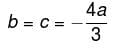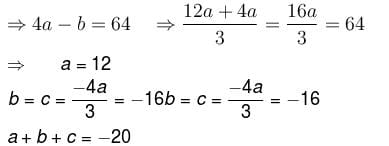*Answer can only contain numeric values
Vector Calculus NAT Level - 2 - Question 3

### Evaluate the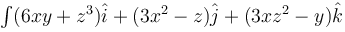along the portion from path (1, 0, 1) to (3, 4, 5) of the curve C, which is the intersection of the surface z2 = x2 + y2 and z = y + 1.

Detailed Solution for Vector Calculus NAT Level - 2 - Question 3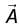can be expressed as gradient of scalar function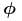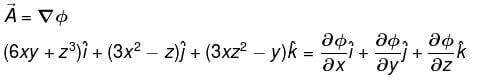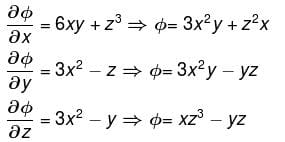written the common term once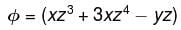The line integral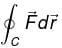along the portion (1, 0, 1) to (3, 4, 5)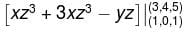= (375 + 108 – 20) – (1)
= 463 – 1
= 462

*Answer can only contain numeric values
Vector Calculus NAT Level - 2 - Question 4

The work done by the force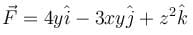in moving a particle over circular path x2 + y2 = 1, z = 0 from (1, 0, 0) to (0, 1, 0) is :

Detailed Solution for Vector Calculus NAT Level - 2 - Question 4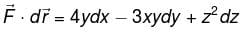on the curve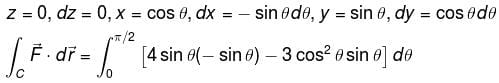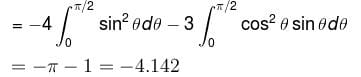*Answer can only contain numeric values
Vector Calculus NAT Level - 2 - Question 5

Evaluate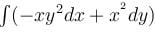where C is the path shown in figure.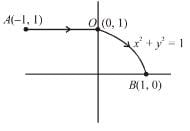Detailed Solution for Vector Calculus NAT Level - 2 - Question 5

Path AO
y
= 1
dy = 0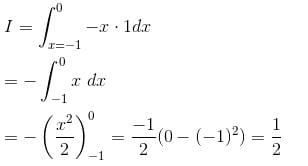Path OB
x
2 + y2 = 1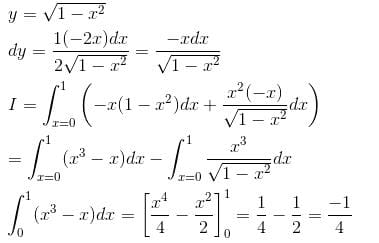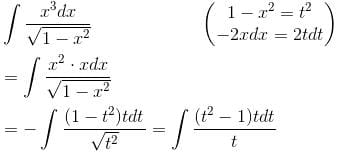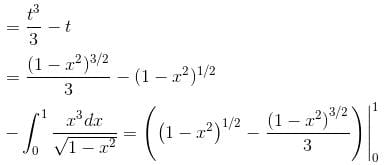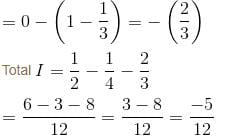= –0.416

*Answer can only contain numeric values
Vector Calculus NAT Level - 2 - Question 6

Let C be any curve x2 + y2 + z2 = 4, z > 0 and the vector field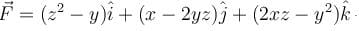find out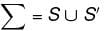(Ans. upto three decimal places)

Detailed Solution for Vector Calculus NAT Level - 2 - Question 6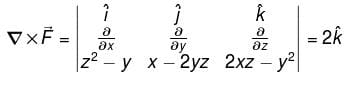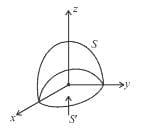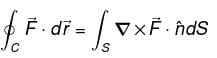Consider a closed surface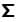consisting of S and S' i.e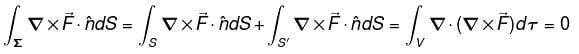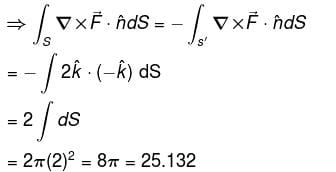*Answer can only contain numeric values
Vector Calculus NAT Level - 2 - Question 7

The value of the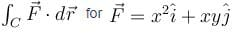and C is the curve y2 = x joining (0, 0) to (1, 1) is (correct upto three decimal places)

Detailed Solution for Vector Calculus NAT Level - 2 - Question 7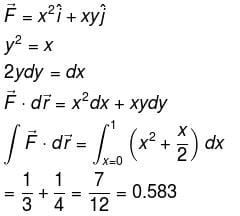*Answer can only contain numeric values
Vector Calculus NAT Level - 2 - Question 8

Find the value of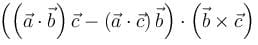Detailed Solution for Vector Calculus NAT Level - 2 - Question 8

Let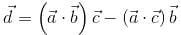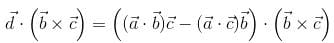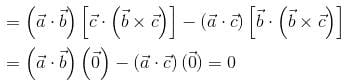*Answer can only contain numeric values
Vector Calculus NAT Level - 2 - Question 9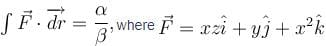along the curve x = sin θ cos θ, y sin2 θ, z = cos θ with θ  increasing from 0 to π/2. Find the value of α + β.

Detailed Solution for Vector Calculus NAT Level - 2 - Question 9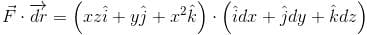xz dx + y dy + xdz
Along the given curve, we have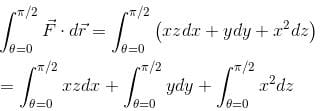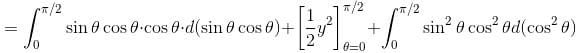Putting values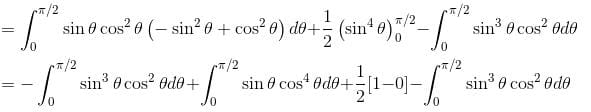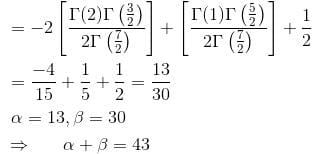*Answer can only contain numeric values
Vector Calculus NAT Level - 2 - Question 10

If f(x, y, z) = x2y + y2z + z2x for all (x, y, x) ∈ R3 and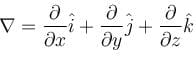then the value of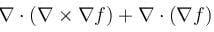at (2, 2, 2) is :

Detailed Solution for Vector Calculus NAT Level - 2 - Question 10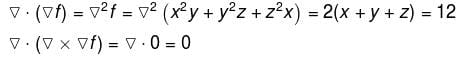Hence,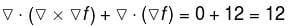## Topic wise Tests for IIT JAM Physics

217 tests
Information about Vector Calculus NAT Level - 2 Page
In this test you can find the Exam questions for Vector Calculus NAT Level - 2 solved & explained in the simplest way possible. Besides giving Questions and answers for Vector Calculus NAT Level - 2, EduRev gives you an ample number of Online tests for practice

## Topic wise Tests for IIT JAM Physics

217 tests(Scan QR code)